## A factory is having problems with production equipment. As a result, 20% of the product wrappings are defective. If 10 products are selected

Question

A factory is having problems with production equipment. As a result, 20% of the product wrappings are defective. If 10 products are selected at random, find the probability of the following:
1. Exactly 3 will have defective wrapping
2. At least 4 will have defective wrapping
3. At most 4 will have defective wrapping
4. At least 2 will have defective wrapping
5. Calculate the mean (i.e. expected value of x)
6. Calculate the standard deviation

in progress 0
6 months 2021-07-31T01:05:38+00:00 1 Answers 4 views 0

1. 0.2013 = 20.12% probability that exactly 3 will have defective wrapping.

2. 0.1209 = 12.09% probability that at least 4 will have defective wrapping.

3. 0.9671 = 96.71% probability that at most 4 will have defective wrapping.

4. 0.6242 = 62.42% probability that at least 2 will have defective wrapping.

5. 2

6. 1.265

Step-by-step explanation:

For each product, there are only two possible outcomes. Either it is defective, or it is not. The probability of a product being defective is independent of any other product, which means that the binomial probability distribution is used to solve this question.

Binomial probability distribution

The binomial probability is the probability of exactly x successes on n repeated trials, and X can only have two outcomes.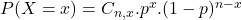In which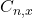is the number of different combinations of x objects from a set of n elements, given by the following formula.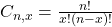And p is the probability of X happening.

20% of the product wrappings are defective.

This means that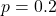10 products are selected at random

This means that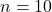1. Exactly 3 will have defective wrapping

This is P(X = 3). So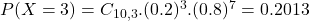0.2013 = 20.12% probability that exactly 3 will have defective wrapping.

2. At least 4 will have defective wrapping

This is: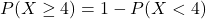In which: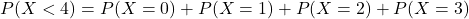So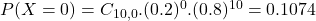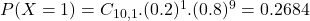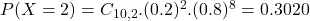Then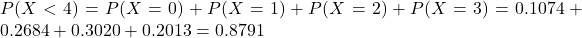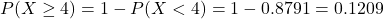0.1209 = 12.09% probability that at least 4 will have defective wrapping.

3. At most 4 will have defective wrapping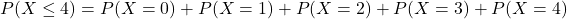In the previous item, the only missing was P(X = 4). So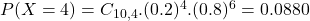So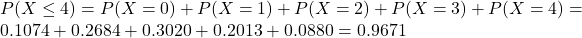0.9671 = 96.71% probability that at most 4 will have defective wrapping.

4. At least 2 will have defective wrapping

This is: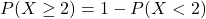In which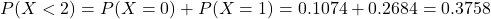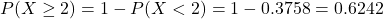0.6242 = 62.42% probability that at least 2 will have defective wrapping.

5. Calculate the mean (i.e. expected value of x)

For the binomial distribution: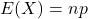So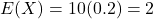The mean is of 2.

6. Calculate the standard deviation

For the binomial distirbution: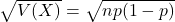In this case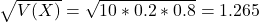The standard deviation is of 1.265.